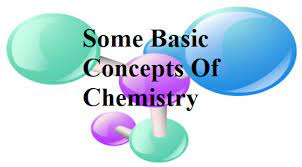# Some Basic Concepts of Chemistry: Composition, Structure, and Properties of Matter

In this quiz, you will find questions related to the basic concepts of chemistry including composition, structure, and properties of matter around us.Start Quiz

As, per the current system, carbon-12 has been taken as the standard for measuring atomic masses.

True False

What is the mass of hydrogen in terms of amu?

1.0020 amu 1.0180 amu 1.0070 amu 1.0080 amu

What is the abbreviation of amu?

Atomic matter unit Atomic mass unified Atomic mass unit At mass unity

Nowadays, “amu” is replaced by ____

u g kg am

A sample of carbon that contains 70% carbon-12 and 30% carbon-14. What do you think is the average atomic mass of this sample?

14.5 14.14 14 12

Elements X and Y combine to form two compounds XY and X2Y. Find the atomic weight of X and Y, if the weight of 0.1 moles of XY is 10g and 0.05 moles of X2Y is 9g

30, 20 80, 20 60, 40 20, 30

Which one will have maximum numbers of water molecules?

18 molecules of water 1.8 grams of water 18 grams of water 18 moles of water

Find the volume of O2 required to burn 1 L of propane completely, measured at 0℃ temperature and 1 atm pressure

10 L 7 L 6 L 5 L

The number of moles present in 6 gms of carbon is:

2 0.5 5 1

The -ve charged particles is called:

Which is not a unit of pressure:

Bar N/m² Kg/m² Torr

What is the normality of a 1 M solution of H3PO4

0.5 N 1.0 N 2.0 N 3.0 N

Which of the following is an example of a heterogeneous substance?

Candle Pieces of copper Table salt Bottled water

Which of the following statements about a compound is incorrect?

A compound retains the physical properties of its constituent elements. The ratio of atoms of different elements in a compound is fixed A compound cannot be separated into its constituent elements by physical methods of separation. A molecule of a compound has atoms of different elements.

Which one of these is not a pure compound?

Sucrose solution H2O2 O3 H2O

Difference in density is the basis of

Gravity separation

Molecular sieving

Ultrafiltration

Molecular attraction

Under the Same Conditions, Nitrogen and Oxygen are Taken in the Same Mass. The Ratio of Their Volumes will be ____.

7 : 8

9 : 2

5 : 6

3 : 5

What is the concentration of nitrate ions if equal volumes of 0.1 M AgNO3 and 0.1 M NaCl are mixed together

0.1 N

0.2 M

0.05 M

0.25 M

The number of atoms present in 0.1 moles of a triatomic gas is

1.806 × 10^23

1.806 × 10^22

3.600 × 10^23

6.026 × 10^22

A gas X has Cp and Cv ratio as 1.4, at NTP 11.2 L of gas X will contain_______ number of atoms.

1.2 × 10^23

3.01 × 10^23

2.01 × 10^23

6.02 × 10^23

Quiz/Test Summary
Title: Some Basic Concepts of Chemistry: Composition, Structure, and Properties of Matter
Questions: 20
Contributed by: